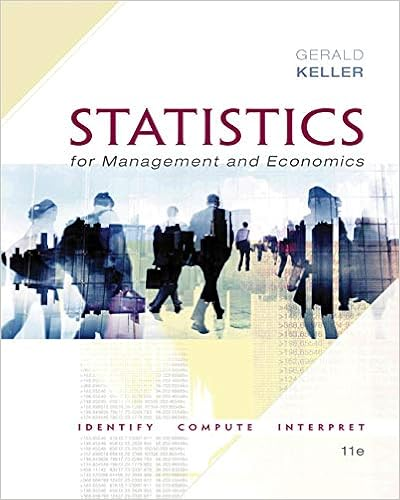# Fill rate e sales e d e d e backorder e d 1 e

• Notes
• yomen1
• 38
• 67% (3) 2 out of 3 people found this document helpful

This preview shows page 22 - 25 out of 38 pages.

##### We have textbook solutions for you!
The document you are viewing contains questions related to this textbook.The document you are viewing contains questions related to this textbook.
Chapter 22 / Exercise 22.8
Statistics for Management and Economics + XLSTAT Bind-in
KellerExpert Verified
fill . rate = E sales E D = E D E backorder E D = 1 E backorder µ 1 . How to choose base stock level S to meet the in-stock probability requirement? Let D F . Given the instock probability, say 97.5%, find S such that P ( D S ) = F ( S ) = 0 . 975 . Hence, we need to find inverse, S = F 1 (0 . 975) . If D ( µ, σ 2 ) , then S = µ + 1 . 96 · σ ; in Excel, S = norm . inv ( 0 . 975 , mu , sigma ) . How to choose base stock level S to hit the fill rate target? First, from the fill rate require- ment fill . rate = E sales / E D = ( µ E back . order) 1 , we find the expected backorder. Then use E back . order = σ +1 L(z) to find z ; from z = S µ +1 σ +1 we find base stock level S . 5.2 EOQ Model Your annual demand for food is stable at rate D . The fixed ordering cost of grocery shopping is K , regardless of quantity you buy; the unit inventory holding cost is h . If every time you buy x amount of food, it will last x/D year. Hence, you need to buy D/x times per year, and your total ordering cost is KD/x . In each cycle, the average inventory is x/ 2 , and hence the total hold cost is hx/ 2 . The main trade-off in determining optimal quantity x is to balance the ordering cost and holding cost. Formally, you have a nonlinear programming problem min x 0 V ( x ) = KD x + hx 2 . The objective function is convex (i.e., V ′′ ( x ) 0 ), hence the necessary and sufficient condition for the optimal solution is V ( x ) = KD x 2 + h 2 = 0 , 22
##### We have textbook solutions for you!
The document you are viewing contains questions related to this textbook.The document you are viewing contains questions related to this textbook.
Chapter 22 / Exercise 22.8
Statistics for Management and Economics + XLSTAT Bind-in
KellerExpert Verified
which yields the economic order quantity (EOQ) x = 2 KD h . 23
6 Week 6 6.1 Risk-Pooling The idea of risk-pooling is based on the correlation of random variables. Consider random vari- ables X , Y , Z . The covariance of X and Y is Cov( X, Y ) = E [( X µ X )( Y µ Y )] = E ( XY ) µ X µ Y . The correlation coefficient of X and Y is ρ = Cov( X,Y ) σ X · σ Y , with 1 ρ 1 . In particular, ρ < 0 indicates negative correlation, ρ > 1 suggests positive correlation, and ρ = 0 means no correlation. 4 In particular, Cov( X, Y ) = ρ · σ X · σ Y , Cov( X, X ) = σ 2 X , and V ar ( X + Y ) = V ar ( X ) + V ar ( Y ) + 2 Cov ( X, Y ) . Suppose two demands X and Y have the same mean µ and standard deviation σ . When they are managed independently , the total standard deviation is 2 σ . When they are managed jointly , we have pooling demand Z = X + Y , with µ Z = E Z = E ( X + Y ) = µ + µ = 2 µ σ 2 Z = V ar ( Z ) = V ar ( X + Y ) = σ 2 + σ 2 + 2 ρσσ = 2(1 + ρ ) σ 2 σ Z = 2(1 + ρ ) · σ. (18) cv Z = µ Z σ Z = 2(1 + ρ ) · σ 2 µ = (1 + ρ ) 2 · σ µ From Eq. (18), we see that as long two demands are not perfectly positively correlated (i.e., ρ < 1 ), pooling always reduces risk (relative to unpooled demands): 2(1 + ρ ) · σ 2 × 2 · σ = 2 σ. 6.2 Optimization In a changing business world, firms often need to made decisions with uncertain outcomes. For example, to plan a promotion, a furniture company needs to stock chairs before customer demand and selling price are fully known. To save cost, a manufacturer needs to buy components overseas, and run production long before customers place their orders. In each case, the decision is made when the future is uncertain—bet before tossing the coin.
•••